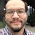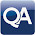## Sunday, November 17, 2013

### HP Prime Programming Tutorial #6: Subroutines

Subroutines

This session will show how routines work in HPPL. Generally, subroutines have be declared before the main program. Declaration is important. The details of the subroutines are after the main program.

Definitely take a look at the example programs to get a better understanding.

SUB Routines for HP Prime

General Syntax:

sub(); //declare subroutines

EXPORT main()
BEGIN
commands go here, including sub()
END;

sub()
BEGIN
commands go here
END;

SUBEXAM

This is just a demonstration of how sub routines work. This program calculates one of two values:

If A is positive, then the program evaluates A. If not, the program values B instead. Where:

A = 2(x-y)/Φ + xy
B = Φ^2

and Φ = 2e^(x+y) - e^(x-y) - e^(y-x)

We will use Φ as the subroutine.

SUB1();

EXPORT SUBEXAM(X,Y)
BEGIN
LOCAL A, B;
A:=(2*(Y-X))/SUB1(X,Y)+X*Y;
B:=(SUB1(X,Y))^2;
IF A>B THEN
RETURN A;
ELSE
RETURN B;
END;
END;

SUB1(X,Y)
BEGIN
RETURN 2*e^(X+Y)-e^(X-Y)-e^(Y-X);
END;

Examples:

SUBEXAM(-4, 1) returns 21998.918189
SUBEXAM(2,3) returns 86283.2797974
SUBEXAM(-5,-6) returns 30.648061288
SUBEXAM(2,-3) returns 21810.6046664

Days Between Dates

DDAYS Using Subroutines for HP Prime: Best for 1901 to 2099

* Remember century years not divisible by 400 are NOT leap years. This program does not take this into account. If any such years are passed, subtract one day for such year manually.

Source: HP 12C Manual - Hewlett Packard

// Declare Subroutines
SUB1();
SUB2();
SUB3();

// Main program
EXPORT DDAYS(m1,d1,y1,m2,d2,y2)
BEGIN
// ΔDYS HP 12C
LOCAL x1, x2, z1, z2;
x1:=SUB1(m1); x2:=SUB1(m2);
z1:=SUB2(m1,y1); z2:=SUB2(m2,y2);
RETURN SUB3(y2,m2,d2,z2,x2)-
SUB3(y1,m1,d1,z1,x1);
END;

SUB1(X)
BEGIN
IF X≤2 THEN
RETURN 0;
ELSE
RETURN IP(.4*X+2.3);
END;
END;

SUB2(X,Y)
BEGIN
IF X≤2 THEN
RETURN Y-1;
ELSE
RETURN Y;
END;
END;

SUB3(Y,M,D,Z,X)
BEGIN
RETURN 365*Y+31*(M-1)+D+IP(Z/4)-X;
END;

(Thanks to Owitte for pointing out my typo)

Examples:
Days Between Dates:

7/3/1985 to 2/28/1995 is 3,527 days

3/14/1977 to 11/17/2013 is 13,397 days

12/10/2010 to 6/30/2014 is 1,298 days

1/5/2015 to 3/19/2227 returns 77,506 BUT this program treats 2100 and 2200 as leap years, which in reality they are not. Subtract 2 to get the correct answer of 77,504 days.

So that is how subroutines work. Please give comments, ask questions, and always thanks to my supporters and readers. Cheers!

Eddie

This blog is property of Edward Shore. 2013

1.Yes your are wright and thanks for post a good topic . your post is

top most in related post of
Eddie's Math and Calculator Blog.

1.Thanks - I am to put additional tutorials regarding the HP Prime this month. Now that things have slowed down between going to a conference at work and Thanksgiving, I'll need to get off my behind.

Eddie

2.Hi,
I checked your program and did not get the right results. I compared your code with the HP12 manual. The following lines need to be changed:

y1:=SUB2(m1,y1); y2:=SUB2(m2,y2);

to
z1:=SUB2(m1,y1); z2:=SUB2(m2,y2);

After this change I got the same results that you posted in your blog.

1.The edit has been done! Thank you so much for letting me know,

Eddie

2.Thank u very much 4 ur excellent PRIME-tutorial!!
Hi Eddie, i should more often take a look into old HP manuals! ;-)
Here's my try of programming the DDAYS Function.
Greetings from Berlin, Germany!
Yours Wolfgang Kuehn.

//---------------------------------------------------------------------------------------
// DJD (Delta Julian Date) is an empty hull for:
// DDAYS Function for HP Prime
// Version 0.8, March 2014
// Wolfgang Kuehn
//
// Delta Days Function: Returns the number of days between two dates
// If the 2nd date is chronologically later, the result is negative
// Range of allowable dates is from October, 15, 1582 to December 31, 9999
// CAS & Home @ algebraic and textbook mode: DDAYS(Y,M,D,Y1,M1,D1)
// example1 DDAYS(2000,1,1,2014,3,17) returns -5189
// example2 DDAYS(1962,10,14,1926,6,1) returns 13284
// CAUTION: @this version there is no recycling of input errors!
// algorythms and formulas are astronomical basics, there are a lot of sources
//---------------------------------------------------------------------------------------

EXPORT DDAYS(Y,M,D,Y1,M1,D1)
BEGIN
IF M <3 THEN
IF M1<3 THEN
RETURN (FLOOR(365.25*(Y-1))+FLOOR(30.6001*(M+13))+FLOOR(((Y-1)/400))-FLOOR(((Y-1)/100))+1720997+D)-(FLOOR(365.25*(Y1-1))+(FLOOR(30.6001*(M1+13))+FLOOR(((Y1-1)/400))-FLOOR(((Y1-

1)/100))+1720997+D1));
ELSE
RETURN (FLOOR(365.25*(Y-1))+FLOOR(30.6001*(M+13))+FLOOR(((Y-1)/400))-FLOOR(((Y-1)/100))+1720997+D)-(FLOOR(365.25*Y1)+FLOOR(30.6001*(M1+1))+FLOOR((Y1/400))-FLOOR((Y1/100))+1720997+D1);
END;
END;
IF M >2 THEN
IF M1 >2 THEN
RETURN (FLOOR(365.25*Y)+FLOOR(30.6001*(M+1))+FLOOR(((Y)/400))-FLOOR(((Y)/100))+1720997+D)-(FLOOR(365.25*Y1)+FLOOR(30.6001*(M1+1))+FLOOR((Y1/400))-FLOOR((Y1/100))+1720997+D1);
ELSE
RETURN (FLOOR(365.25*(Y))+FLOOR(30.6001*(M+1))+FLOOR((Y/400))-FLOOR((Y/100))+1720997+D)-(FLOOR(365.25*(Y1-1))+FLOOR(30.6001*(M1+13))+FLOOR(((Y1-1)/400))-FLOOR(((Y1-1)/100))+1720997+D1);
END;
END;
END;

3.Thank you for both your compliment and the program!

Eddie

3.It was so nice article.I was really satisified by seeing this article and we are also giving QA online training.The QA online training is one of the best QA online training institute in worldwide.

4.Hi Eddie,

I also like calculators. HP Prime is a good one.
Thank you for this beautiful site.

I think there is an error in sub1.
For the formula 0.4*X+2.3 (it is interesting also)
month 4 -> 121 (it should be 120)
month 6 -> 182 (it should be 181)
month 9 -> 274 (it should be 273)
month 11 -> 335 (it should be 334)

I try different values for the formula.
0.44*X+2.4 seems good to me.

Rick

5.I think this is one of the most significant information for me.And i’m glad reading your article.But should remark on some general things, the web site style is perfect,the articles is really great : D. Good job…
IWIN89

### Retro Review: Radio Shack EC-2024

Retro Review:  Radio Shack EC-2024 Quick Facts Company:  Radio Shack Years in Production: Around 1994 Original Price: \$24.99 Bat...